# Array Declaration and Initialization

## Array: Declaration and Initialization

In this article, I am going to give you a brief introduction to Array Declaration and Initialization. As part of this article, we are going to discuss the following pointers.

1. What is an array?
2. How to declare and initialize an array?
3. What are the different methods for accessing an array?
##### Understanding Simple Variables:

Before understanding the array, let us first understand simple variables. Variables are supported by every programming language and the variables will have the same data type. For example, if we want to declare a variable of type integer, whose name is x and in this, if we want to store the value 10 then we need to write something like below in c language.

int x = 10;

Then we know very well that at runtime variable x will get some memory. If we assume that integer takes two bytes of memory, then two bytes of memory will be allocated for x. Let say the 1st-byte address is 100 and the next byte address is 101 and together in these two bytes the value 10 will be stored as shown in the below image. This type of variable is a single value variable i.e. they just store one single value.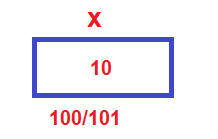The single value variable is also called a scalar variable. Then what is an array?

##### What is an Array?

In the array variable, we can store multiple values that are a list of values or a set of values. So, we can define an array as a collection of elements and all the elements are of the same type. In simple words, the array is a collection of similar data elements grouped under one name.

Suppose instead of 1 value, if we want to store a list of values, then we can declare an array. Let us say we want to create an integer type array; whose name is A and the size is 5 and in C language the following line of code does the same.

int A;

In this array, we can store 5 integers and the indices will be 0 1 2 3 4 as shown in the below image. And here we can store 5 values.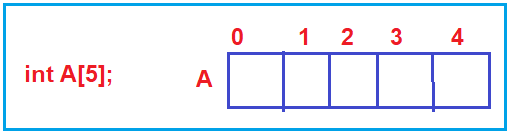The array variable is also called Vector variables i.e. having some dimension.

##### Difference between Scalar and Vector Variable:

In the case of scalar variable i.e. x, we can store a single value in it and the memory is 2 bytes. On the other hand, A is a vector variable and in this variable, we can store 5 integer values and the memory it will occupy is 10 bytes (we are considering integer takes 2 bytes). For example, the first-byte address is 201 and then 202, 203, up to 210. The most important point that you need to remember is that the memory will be allocated continuously.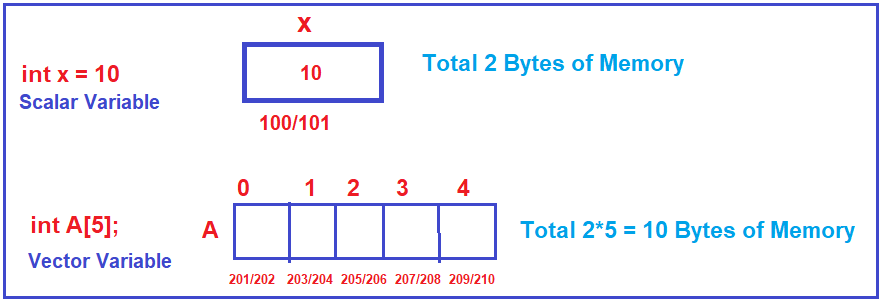All the five integers will be having the same name i.e. A. Then how we can differentiate all those five integers. We can differentiate them with the index which will start from 0 up to 1. So, A means the first integer, A means the second integer and A means the fifth integer. So, we can access them or differentiate them through indexes.

Suppose we want to store a value 15 at index 2 then we can do the same using the below line of code in most programming languages.

A=15;

Once you write the above code, then it will store 15 in the index position 2 on the array as shown below.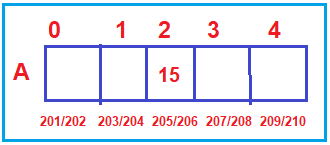So, using the name and the index we can access any of those integers or any of those elements.

The array is a collection of elements or a list of elements that are of the same type and this concept is supported by every programming language as a basic feature. With this keep in mind let us proceed and understand what are the different ways to declare an array.

##### Declarations and Initialization of Array:
###### First method:

Declare an array of some size without Initializing. As you can see in the below image, we are declaring an integer array with the name A of size 5, then this will allocate the memory space for 5 integers starting from indexes 0 to 4. As we have not initialized the array with any values, so by default it will store garbage values.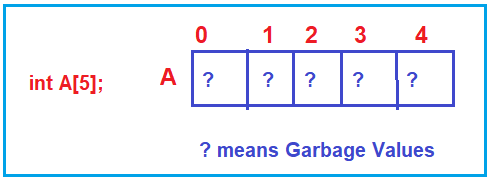Note: When the array is not initialized with any values then by default it will store garbage or unknown or random values in it.

###### Second method:

We will declare an array of some size and also, initialize with values. As you can see in the below image, we created an array with the name A and size 5 as well as we initialize the array with five elements. In this case, the array will be created during the runtime and all the values will be initialized at that time.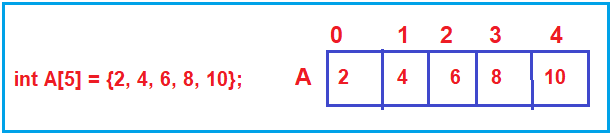Note: The above example is the declaration + initialization

###### Third method:

We will declare an array of some size but we will initialize only a few values. As you can see in the below image, we have created an array with the name A and size 5 and initialize it only with three elements. In this case, the rest values will be initialized with 0 by default.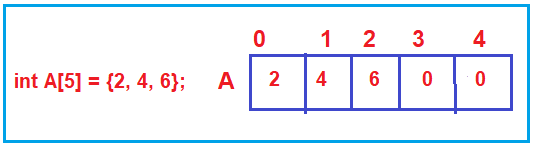Here, the array is initialized with three values, so the first three indexes are initialized with the values 2,4, and 6, and the last two index positions are initialized with 0. So, the point that you need to remember is, once the initialization process starts it will try to initialize all the elements and if values are not present then it will initialize with 0.

###### Fourth method:

We will declare an array of some size and we will assign only 0 as the first element, the rest of the elements are also filled with 0. As you observe in the below image, array A is created with size 5 and initialized with only one value i.e. 0 and in this case, the rest four indexes are also initialized with 0.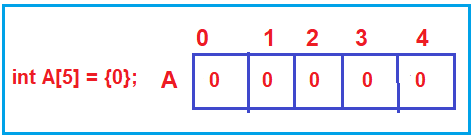###### Fifth Method:

We will not mention any size but initialize with elements. As you can see in the below image, we created an array with the name A without mentioning the size but initializing the array with five elements. Depending on the number of elements we have mentioned in the initialization list, the array size will be the same as that one. So, an array with size 5 will be created in this case and it will be initialized with all those values.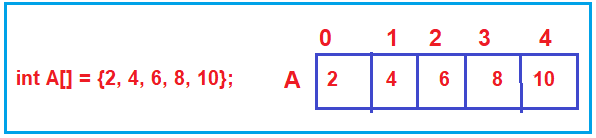So, these are the various ways of declaration and initialization of an array. This is there in both C and C++. Now I hope you understand how to declare and initialize an array. Let us proceed further and understand how to access the elements of an array.

##### Accessing Array Elements:

Let say we have an integer array of size 5 and this array is initialized with five integers as shown in the below image.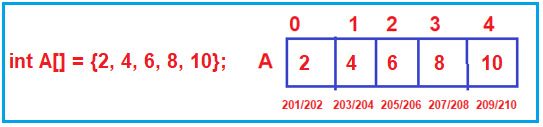Now, how to access those elements. Suppose we want to print the value that is present at index 2, then we can do the same using A as shown below,

printf(“%d”, A);

Suppose we want to print the value that is present at index 3, then we can do the same using A as shown below,

printf(“%d”, A);

Note: The point that you need to remember is, we can access all the array elements just by changing the index.

If we have to traverse through an array (traversing means visiting all elements once), then we can take the help of a loop which will help us to change the indexes from 0 to 4 and that will allow us to access all the elements by traversing through those elements as shown below. ‘for’ loop is used for accessing the elements in an array.

int A = {2, 4, 6, 8, 10};
for(i=0;i<5;i++)
{
printf(“%d”, A[i]);
}

Note: for loop is used for traversing through all the elements of an array. And the elements can be accessed with the name and index of an array.

Next let us see what are the different ways of accessing a particular element in an array,

1. printf(“%d”, A); It will print the element which is present at index position 2.
2. printf(“%d”, 2[A]); We can also access the element using index outside and the name of an array inside subscript.
3. printf(“%d”, *(A+2)); We can also access the element using pointer arithmetic.

Note: The array element can be accessed by using the subscript [] that is an index as well as they can be accessed with the help of pointer arithmetically.

##### Demonstration of Array Declaration and Initialization:

Let us see some programming examples to understand the Declaration and Initialization.

###### Example:

In the below example, we have declared and initialize five different arrays.

1. In array A, we have only declared but not initialized, in this case, it will store some garbage values and that what you can see when you print the elements (Sometimes you may see the values are 0, but they are also garbage).
2. In array B, we have declared and initialized the array with five elements and those elements you can when you traverse the array (traverse means accessing each element).
3. In array C, we have mentioned the size as 5, but initialized with 2 elements, in this case when you access the array elements, the first two values you will get as expected but the last three values you will get the default values based on the data type. As the data type, we used here is int, so you will get the default value of int i.e. 0.
4. In array D, we declared the array size as 5 but initialize the array only with one element and that is too with the value 0. In this case, the rest four elements are also initialized with 0.
5. In array E, we have not mentioned the array size but initialized with five elements. In this case size of the array will same as the number of elements we initialized i.e. 5. When we access the array, we will get the elements as expected.
```#include <stdio.h>
#include <stdlib.h>
int main()
{
//Declare array of some size without Initialization
int A;
printf(" A Array Elements : ");
for(int i = 0; i <5; i++)
{
printf("%d ",A[i]);
}

//Declare an array of  size 5 and also Initialize the array with 5 values
int B={1, 2, 3, 4, 5};
printf("\n B Array Elements : ");
for(int i = 0; i <5; i++)
{
printf("%d ",B[i]);
}

//Declare an array of size 5 but Initialize only few values
int C={2, 4};
printf("\n C Array Elements : ");
for(int i = 0; i <5; i++)
{
printf("%d ",C[i]);
}

//Declare an array of size 5 and assign only 0 as the first element
int D={0};
printf("\n D Array Elements : ");
for(int i = 0; i <5; i++)
{
printf("%d ",D[i]);
}

// We will not mention any size but will initialize the array with some elements
int E[]={1,2,3,4,5,6};
printf("\n E Array Elements : ");
for(int i = 0; i <5; i++)
{
printf("%d ",E[i]);
}

return 0;
}
```

Output: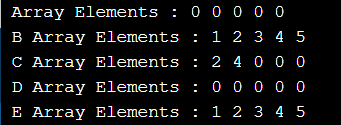##### Example: Array address are in contiguous

As we already discussed earlier, the array stores the elements in the contiguous memory location. Let us see an example and proof that the addresses are contiguous.

```#include <stdio.h>
#include <stdlib.h>

int main()
{
int A;
for(int i = 0; i <5; i++)
{
printf(" %u \n",&A[i]);
}

return 0;
}
```

Output: As you can see in the below output each index takes 4 bytes and all indexes are contiguous and hence it proofs the array stores the elements in a contiguous memory location.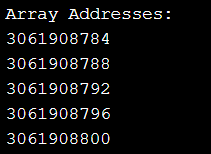In the next article, I am going to discuss the Static VS Dynamic Array. Here, in this article, I just give an overview of Array Declaration and Initialization and I hope you enjoy this Array Declaration and Initialization article.